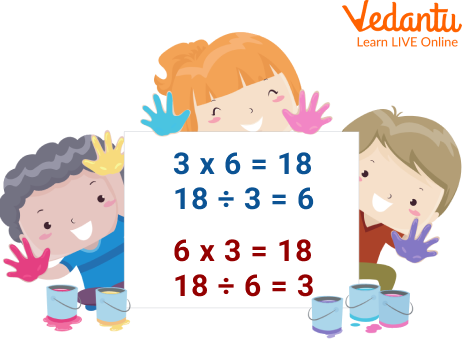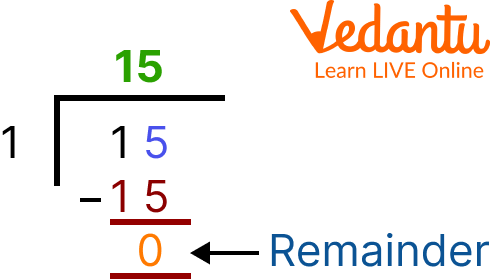Courses
Courses for Kids
Free study material
Free LIVE classes
More

# Multiplication and Division Facts## An Introduction to Multiplication and Division

Multiplication and division are basic knowledge for school students. Multiplication is the process of calculating the product of two or more numbers. It is a basic arithmetic operation that is frequently used in everyday life. When we need to combine groups of equivalent size, we employ multiplication. Division is the separating of a large group into smaller groups so that each group has an equal number of things. In Mathematics, it is an operation used for equal grouping and equal sharing. In this article, we will introduce you to some well-known multiplication and division facts.

## What are Multiplication and Division Facts?

The numbers that are being multiplied are referred to as factors, and the outcome of the multiplication is referred to as the product.

In division, the dividend is the number being divided, the divisor is the number dividing it, and the quotient is the outcome of the division.Multiplication and Division

## Division and Multiplication-related Facts

• You can write a multiplication fact simply by writing out a multiplication calculation. For example: 12 × 12 = 144, 3 × 6 = 18 and 8 × 5 = 40

• You can also write multiplication facts using different methods of multiplication. For example, repeated addition is a way of finding multiplication facts by repeatedly adding a number until you reach the answer. 2 × 5 is the same as 2 + 2 + 2 + 2 + 2 = 10. The multiplication fact in that case is 10.

• If the dividend is ‘zero’ then any number as a divisor will give the quotient as ‘zero’.

Example: If ‘zero’ sweets are to be distributed among 8 children, naturally no one will get any sweets.

• If the divisor is ‘1’ then any dividend will have the quotient equal to itself.

Example: There are 15 sweets; each child is to get 1 sweet. How many children get sweets?Division of 15 by 1

## Multiplication and Division Drills

Q1. At a zoo, there are 45 deer. Find out the total number of legs of all the deer.

Ans: There are a total of 45 deer. We know that each deer will have 4 legs.

Therefore, the total number of legs deer will have will be equal to the:

$= 45 \times 4$
= 180.

Hence, 180 will be the total number of legs of all the deer.

Q2. Write two multiplication for each division:

(i) 15 ÷ 3 = 5

Ans: The two multiplication for it will be: 5 × 3 = 15 and 3 × 5 = 15

(ii) 3 ÷ 3 = 1

Ans: The two multiplication for it will be: 1 × 3 = 3 and 3 × 1 = 3

## Practice Questions

Q1. Write two multiplication for each division:

(i) 21 ÷ 3 = 7

(ii) 30 ÷ 3 = 10

(iii) 33 ÷ 3 = 11

Ans:

(i) 7 × 3 = 21 and 3 × 7 = 21

(ii) 10 × 3 = 30 and 3 × 10 = 30

(iii) 11 × 3 = 33 and 3 × 11 = 33

Q2. Write two divisions for each multiplication:

(i) 6 × 3 = 18 (Ans: 18 ÷ 3 = 6 and 18 ÷ 6 = 3)

(ii) 2 × 3 = 6 (Ans: 6 ÷ 2 = 3 and 6 ÷ 3 = 2)

(iii) 9 × 3 = 27 (Ans: 27 ÷ 9 = 3 and 27 ÷ 3 = 9)

(iv) 12 × 3 = 36 (Ans: 36 ÷ 3 = 12 and 36 ÷ 12 = 3)

(v) 8 × 3 = 24 (Ans: 24 ÷ 3 = 8 and 24 ÷ 8 = 3)

## Summary

In this article, we have learned about multiplication which is a mathematical operation that conveys the fundamental concept of repeated addition of the same integer. Later, we also learned about division sentences, as it is a basic mathematical operation in which a bigger number is divided into smaller groups with the same number of components. In the end, we have seen some practice problems based on the facts. We hope you have a clear picture of the facts on multiplication and division. Practise further to enhance your understanding of the topic.

Last updated date: 20th Sep 2023
Total views: 89.4k
Views today: 2.89k

## FAQs on Multiplication and Division Facts

1. What are the 3 types of division?

The methods of division are of three types according to the difficulty level. These are the chunking method or division by repeated subtraction, short division method or bus stop method, and long division method.

2. What are the parts of multiplication?

The different parts of multiplication are expressed as follows. Let us understand this with an example: 6 × 4 = 24.

• Multiplicand (Factor): Multiplicand is the first number. In this case, 6 is the multiplicand.

• Multiplier (Factor): Multiplier is the second number. In this case, 4 is the multiplier.

• Product: The final result after multiplying the multiplicand and multiplier. In this example, 24 is the product.

• Multiplication Symbol: '×' (which connects the entire expression)

3. How does multiplication work in real life?

In our daily lives, we frequently use multiplication. For example, we may calculate the price of things based on the rate per quantity, discover the correct quantity of an ingredient to use in cooking, calculate the value of numerous items when the value of one item is known, and so on.